Courses

# Mining Engineering - (MN) 2018 2018 GATE Paper (Practice Test)

## 65 Questions MCQ Test GATE Past Year Papers for Practice (All Branches) | Mining Engineering - (MN) 2018 2018 GATE Paper (Practice Test)

Description
This mock test of Mining Engineering - (MN) 2018 2018 GATE Paper (Practice Test) for GATE helps you for every GATE entrance exam. This contains 65 Multiple Choice Questions for GATE Mining Engineering - (MN) 2018 2018 GATE Paper (Practice Test) (mcq) to study with solutions a complete question bank. The solved questions answers in this Mining Engineering - (MN) 2018 2018 GATE Paper (Practice Test) quiz give you a good mix of easy questions and tough questions. GATE students definitely take this Mining Engineering - (MN) 2018 2018 GATE Paper (Practice Test) exercise for a better result in the exam. You can find other Mining Engineering - (MN) 2018 2018 GATE Paper (Practice Test) extra questions, long questions & short questions for GATE on EduRev as well by searching above.
QUESTION: 1

Solution:
QUESTION: 2

Solution:
QUESTION: 3

### The area of a square is ��. What is the area of the circle which has the diagonal of the square as its diameter?

Solution:
QUESTION: 4

What would be the smallest natural number which when divided either by 20 or by 42 or by 76 leaves a remainder of 7 in each case?

Solution:
QUESTION: 5

What is the missing number in the following sequence?

2,  12,  60,  240,  720,  1440,  _____,  0

Solution:
QUESTION: 6

In appreciation of the social improvements completed in a town, a wealthy philanthropist decided to gift Rs 750 to each male senior citizen in the town and Rs 1000 to each female senior citizen. Altogether, there were 300 senior citizens eligible for this gift. However, only 8/9th of the eligible men and 2/3rd of the eligible women claimed the gift. How much money (in Rupees) did the philanthropist give away in total?

Solution:
QUESTION: 7

If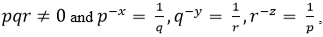what is the value of the product XYZ?

Solution:
QUESTION: 8

In a party, 60% of the invited guests are male and 40% are female. If 80% of the invited guests attended the party and if all the invited female guests attended, what would be the ratio of males to females among the attendees in the party?

Solution:
QUESTION: 9

In the figure below, ∠DEC + ∠BFC is equal to ____________ .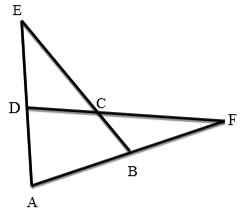Solution:
QUESTION: 10

A six sided unbiased die with four green faces and two red faces is rolled seven times. Which of the following combinations is the most likely outcome of the experiment?

Solution:
QUESTION: 11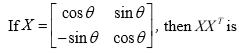Solution:
QUESTION: 12

The values of x satisfying the following condition are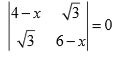Solution:
QUESTION: 13

An azimuth of 330° corresponds to a quadrant bearing of

Solution:
QUESTION: 14

Tri-cone drill bit is a type of

Solution:
QUESTION: 15

Exposure of weak roof in junctions of a development district in a coal mine can be decreased by

Solution:
QUESTION: 16

The property that CANNOT be determined from uniaxial compressive strength test of a rock sample fitted with strain gauges is

Solution:
QUESTION: 17

The radial stress concentration around a long circular tunnel excavated in rock is given by the curve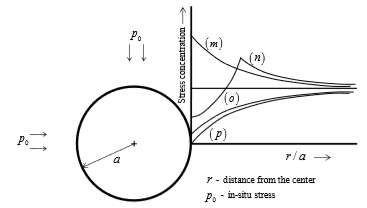Solution:
QUESTION: 18

Ward-Leonard system is provided in the winding system in order to restrict

Solution:
QUESTION: 19

The equipment NOT related to extraction of coal from longwall face operation is

Solution:
QUESTION: 20

The correct figure depicting the extraction of contiguous seams in bord and pillar working is indicated by

Solution:
QUESTION: 21

The significance of ‘potentially explosive mixture’ in Coward flammability diagram is -

Solution:
QUESTION: 22

Considering ‘I’ as ‘intake’ and ‘R’ as ‘return’, the ventilation symbol for the shaft-bottom air-lock in a coal mine is

Solution:
QUESTION: 23

From a coal seam of a mine 1000 tonnes of coal is produced per day. The seam has inflammable gas emission rate of 14000 m3 per day. Percentage of inflammable gas in general body of air is 0.14. The gassiness of the seam is

Solution:
QUESTION: 24

The temperature profiles and the plume patterns that are most likely to result are given in the figures. The dotted line represents ‘adiabatic lapse rate’ and the bold line represents ‘environmental lapse rate’. The WRONG combination is

Solution:
QUESTION: 25

The inventory pattern shown does NOT represent the following.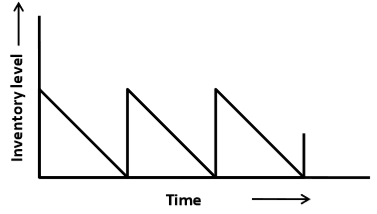Solution:
QUESTION: 26

The figure depicts a transportation problem along with the solution. The correct statement is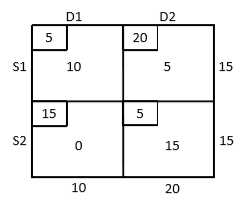Solution:
QUESTION: 27

The degree of the differential equation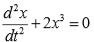is ________________-.

Solution:
QUESTION: 28

The slope of the line connecting the points (20, 6) and (40, 8) is ______________.

Solution:
QUESTION: 29

Two contours of RL 60 m and 70 m are separated by 34 m measured along dip of the seam. The dip of the seam in degree is ________________.

Solution:
QUESTION: 30

The RL of the initial station is 200 m. If ∑ BS = 1.54 m and ∑ FS = 0.45 m , then the RL of the last station in m is ________________.

Solution:
QUESTION: 31

For the conveyor belt drive shown, the tension on the tight side (T1 ) is double that of the slack side. The coefficient of friction between belt and drum is 0.21. The minimum angle of lap (in degree) to avoid slippage of the belt is ________________.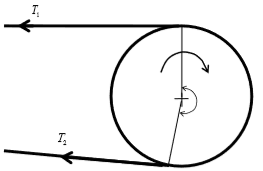Solution:
QUESTION: 32

In a bord and pillar development district, 6 headings driven along the strike direction are surrounded by panel barriers on the dip and rise sides. The maximum possible number of faces for the panel is ___________________.

Solution:
QUESTION: 33

A roadway in a mine, a single light source as shown, emits light uniformly in all directions. The floor level illumination at station A is 20 lux. The floor level illumination at a point B in lux is ______________.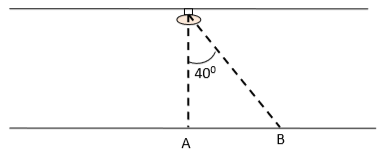Solution:
QUESTION: 34

A system of two identical components connected in series has reliability of 0.25. The reliability of each component is _______________.

Solution:
QUESTION: 35

The operational status of an HEMM in a shift is shown in the diagram. The availability of the machine in % is _____________________.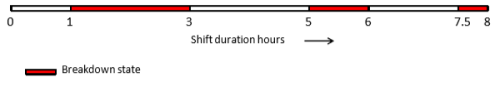Solution:
QUESTION: 36

If c is a constant, the solution of the differential equation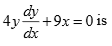is

Solution:
QUESTION: 37

Match the following blasting elements with the corresponding initiators.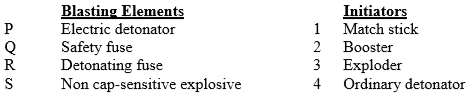Solution:
QUESTION: 38

The following plot is developed for a rock type after a series of triaxial tests. The uniaxial compressive strength and tensile strength of the rock type, respectively, in MPa, are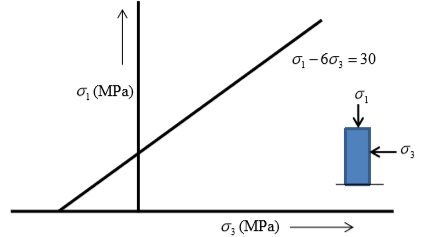Solution:
QUESTION: 39

Match the following in the context of environmental management.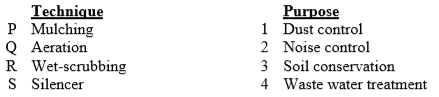Solution:
QUESTION: 40

A panel in coal mine produces 400 tonne per day. The number of persons employed in each of the three shifts is 110, 130 and 120. As per CMR, the minimum quantity of air that needs to be circulated at the last ventilation connection of the panel in m3/min is

Solution:
QUESTION: 41

A right conical iron ore stack on level ground of height 10 m has 60% Fe. The height of the conical stack is extended up to 20 m using iron ore of 50% Fe. The angle of repose of iron ore is 38o. The mean grade of the final stack in % Fe is ___________________.

Solution:
QUESTION: 42

The feasible region of a linear programming problem is shown in the figure. The maximum value of the objective function Z = 4 X+3Y is ____________________.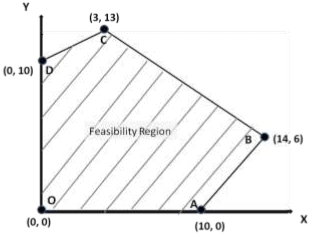Solution:
QUESTION: 43

Cash flow of a project of duration 4 years is shown. The uniform income ‘R’, in Rs. crores, at the end of 2nd, 3rd and 4th years for an internal rate of return of 10% is ______________.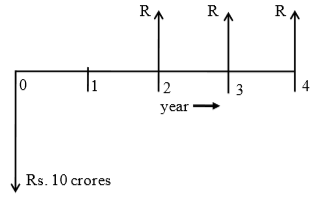Solution:
QUESTION: 44

The grade values of alumina at three sample locations (A, B and C) in a bauxite deposit are as shown. Using the ‘inverse distance’ method, the computed grade in % alumina at location ‘Z’ is ___________________.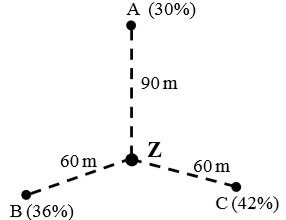Solution:
QUESTION: 45

Economic feasibility of two methods is examined for meeting a targeted mine production. On a yearly basis, the following cost parameters (Rs in crores) are applicable for these methods.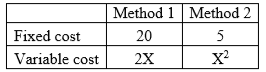The annual rate of production, ‘X’ in million tonnes for which both the methods will yield the same operating cost is ___________________.

Solution:
QUESTION: 46

A person standing 50 m away from an HEMM experiences 90 dB sound pressure level. If the person moves to a new location that is 70 m away from the HEMM, the sound pressure level experienced by the person, in dB, becomes _________________.

Solution:
QUESTION: 47

A portion of a ventilation system has two splits as shown. Split ‘A’ has a resistance of 0.2 Ns2/m8 and a regulator of size 2.0 m2. The resistance of split ‘B’ in Ns2/m8 is ________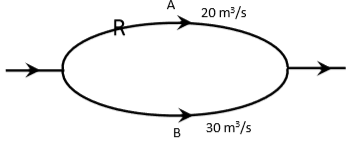Solution:
QUESTION: 48

Air enters a bord and pillar panel at 10 ppm CO and 20.78% O2. The air at the panel return has 80 ppm CO and 20.52% O2. The ‘Graham’s ratio’ for the status of fire in the panel in % is __________________.

Solution:
QUESTION: 49

In a sublevel stope, 20 m high excavation is made with ring drilling in a 6 m wide orebody.  In an effective shift time of 6 hrs, three rings are blasted resulting in 9 m extraction length. LHDs of 5.0 m3 bucket capacity and cycle time of 5 minutes are used for ore transportation. The number of LHDs required for this operation is _________________.

Solution:
QUESTION: 50

A coal seam lying at a depth of 200 m is developed by bord and pillar method. Pillars are 30 m centre to centre with a gallery width of 4 m. Unit weight of the overlying strata is 28 kN/m3. If the pillar strength is 9.32 MPa, the factor of safety of pillar is ____________.

Solution:
QUESTION: 51

Following information about a longwall retreating panel is given.
Panel length = 1800 m
Face length = 150 m
Depth of web = 0.6 m
Shearer travels at a speed of 1.5 m/min along the face.
Each cutting cycle requires a non-operational time of 2 h 20 min. The panel is fully extractable. The number of working days required to extract the panel is ______________.

Solution:
QUESTION: 52

In a direct rope haulage operating along a 1500 m long incline, 180 tonne of coal is hauled in 7 hours. The average rope speed is 7.5 km/h. The set changing time for the tubs is 2 minutes each at the top and bottom of the incline. If the tub capacity is 1.0 tonne, the number of tubs in the set is __________________.

Solution:
QUESTION: 53

An SDL of 1.0 tonne capacity operates with a cycle time of 6 minutes. The dimension of the face is 4 m x 3 m. Five blasts are conducted per shift with an average pull of 1.2 m. If the density of blasted coal is 0.8 tonne/m3, the time required by the SDL in the shift to lift all the prepared coal in hours is _________________.

Solution:
QUESTION: 54

A force diagram is shown below. Considering clockwise moments to be positive, the resultant moment about A in Nm is _________________.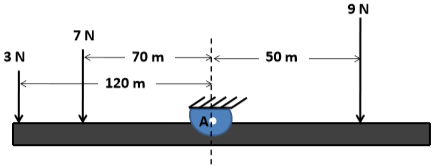Solution:
QUESTION: 55

The experiment to determine permeability of a soil sample is illustrated below. The crosssectional area of the sample is 20.0 cm2. The permeability of the soil sample in cm/s is ______________.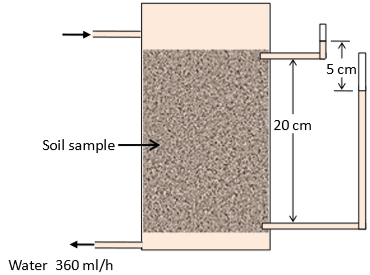Solution:
QUESTION: 56

In underground face blasting, the pull is found to be 10% less than the drillhole length. The headings of 3.6 m width are supported by prop density of 1.44/m2. The hole length is 1.5 m, and 6 rounds of blasting are done in the panel per shift. The number of props to be erected in a shift is ____________________.

Solution:
QUESTION: 57

The immediate roof of a mine is supported by bolts of length 1.5 m, arranged in 1.2 m x 1.2 m grid pattern. If the unit weight of roof rock is 2.25 tonne/m3 and load carrying capacity of each bolt is 7.5 tonne, the factor of safety of the support system is _______________.

Solution:
QUESTION: 58

In a level terrain, a vertical orebody of 20 m uniform width is worked by surface mining method. The density of the ore is 2.5 tonne/m3. The ultimate pit has a depth of 60 m, width of 20 m at pit bottom, and a pit slope of 45o. The overall stripping ratio for this condition in m3/tonne is ____________________.

Solution:
QUESTION: 59

A 20 m long steel tape used for survey is found to be short by 10 cm. If the area measured with the steel tape is 5000 m2, the actual area in m2 is __________________.

Solution:
QUESTION: 60

The following figure shows the designed blast pattern of a bench. The explosive column is charged at 18 kg/m. If the unit weight of the blasted material is 2.5 tonne/m3, the powder factor for the blast in tonne/kg is _______________.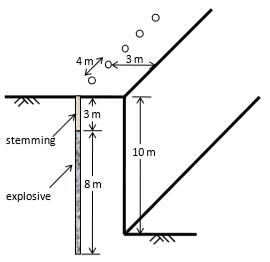Solution:
QUESTION: 61

Two points on the equator have longitudes 55oE and 25oW. Considering radius of earth as 6400 km, the distance between the two points in km is ________________.

Solution:
QUESTION: 62

Sum of the series 5, 10, 15, ……….., 500 is __________________.

Solution:
QUESTION: 63

The sample standard deviation for the following set of observations is _______________. 40, 45, 50 and 55

Solution:
QUESTION: 64

For the given function f ( x, y ) = ( 3 + x ) ( 4 + y ) , the value of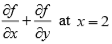and y= 1 is_____________________.

Solution:
QUESTION: 65

Given y = x 2 + x + 6 , the value of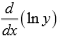at x = 2 is ________________.

Solution: